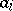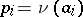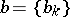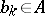# Bernoulli automorphism

(diff) ← Older revision | Latest revision (diff) | Newer revision → (diff)

An automorphism of a measure space, which describes Bernoulli trials and their generalization — a sequence of independent trials with the same result and with the same probability distribution.

Letbe the collection of all possible outcomes of a trial, and let the probability of the eventbe given by the measure; for a countable set, denote its elements byand their probabilities by. The phase space of a Bernoulli automorphism is the direct product of a countable number of copies of the set, i.e. the points of the phase space are infinite sequences, whereruns through the set of integers and each. The transformationconsists in shifting all members of each sequence one place to the left:. The measureis defined as the direct product of a countable number of measures; thus ifis countable, thenIn this case, the entropy of the Bernoulli automorphism is.

In ergodic theory, Bernoulli automorphisms (or, more exactly, the cascades generated by iteration of them) play the role of a standard example of a dynamical system, the behaviour of which displays statistical features. A Bernoulli automorphism is a-automorphism but there exist-automorphisms which are metrically non-isomorphic to a Bernoulli automorphism, even though many-automorphisms are metrically isomorphic to a Bernoulli automorphism. Two Bernoulli automorphisms are metrically isomorphic if and only if they have the same entropy . A Bernoulli automorphism is a quotient automorphism of any ergodic automorphism of a Lebesgue space with a larger entropy .

How to Cite This Entry:
Bernoulli automorphism. Encyclopedia of Mathematics. URL: http://encyclopediaofmath.org/index.php?title=Bernoulli_automorphism&oldid=17876
This article was adapted from an original article by D.V. Anosov (originator), which appeared in Encyclopedia of Mathematics - ISBN 1402006098. See original article